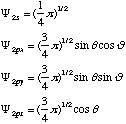1. Imagine we have three atoms of sp2-hybridized carbon side by side. The unhybridized p orbitals of these atoms are parallel to each other. One of the carbons does not have four bonds, but instead has a positive charge located in its unhybridized p orbital. This configuration is called an "allyl cation."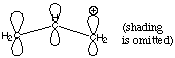A. Why is the positive charge located in the unhybridized 2p orbital instead of one of the sp2 orbitals?

B. How many electrons are located in the three p orbitals (the p system) depicted above?

C. Ignoring the skeletal framework of this molecular ion and the position of the positive charge, add and subtract the wavefunctions of the three p orbitals to construct a molecular orbital diagram describing the situation. Be sure to give your molecular orbitals the correct energetic order.

D. Each of your molecular orbitals can accomodate two electrons. Which orbitals are filled? What does this tell you about the electron density in this allyl cation system?

E. Where is the positive charge in the allyl cation?

2. Before 1913, scientists were unable to measure the atomic numbers of elements directly. Therefore, the elements of the periodic table were ordered by their atomic masses.

A. In some cases, the ordering of elements by atomic mass does not match their order by atomic number. Why?

B. X-rays are emmitted when an electron drops from the 2s orbital of an atom to that atom's 1s orbital. Such radiation is called "Ka x-ray radiation." Which would have a larger energy, the Ka x-rays emmitted by Co or the Ka x-rays emmitted by Ni? Briefly explain your answer.

C. In 1913, a young British scientist named Henry Mosely used Ka x-rays emmission to put the elements of the periodic table into their proper order by atomic number. How did he do it? Why didn't atomic mass make a difference?

3.* (1997 F 10) The NH radical is a highly reactive gas-phase species. Use the LCAO-MO theory to describe its electronic structure. Here the H(1s) atomic orbital is combined with the N(2p) atomic orbital. The ionization potential of H 1s is 13.6 eV and that of N 1s22s22p3 is 14.5 eV. Answer the following questions:

A. Draw an energy level diagram of the MO’s in NH arising from combining the 1s atomic orbital of H with the three 2p atomic orbitals of N. Indicate how the four valence electrons of NH occupy these orbitals.

B. What is the multiplicity of NH in its ground electronic state? Do you expect NH to be paramagnetic or diamagnetic?

C. Draw pictures of the s and s* orbitals of NH showing the charge distribution relative to the N and H atomic centers.

D. Draw pictures of the valence orbitals of NH showing the charge distribution relative to the N and H atomic centers.

E. The ionization potential of NH has been determined to be 13.63eV. Is this value expected by you based on LCAO-MO theory? Explain.

4.* (1997 1 7) Consider the diatomic molecule NF. Draw its molecular orbital energy diagram.

A. Using the LCAO-MO scheme, indicate the ground-state MO description for NF, i.e. complete the following: 1(s)2 . . .

B. What is the multiplicity of the ground state of NF?

C. Do you expect NF to be diamagnetic or paramagnetic? Why?

D. The ionization potential of N is 14.54 eV and that for F is 17.42 eV. Do you expect the ionization potential of NF to be greater than that of F, closer to N than F, closer to F than N, or less than N? Explain.

5.* (1996 F 7) Four oxidation state of molecular dioxygen are known: [O2]n where n= 0, +1, -1, -2. They are dioxygen, dioxygen cation, superoxide anion, and peroxide dianion, respectively. The O-O distances and infrared stretching frequencies have been measured for these four oxidation states.

A. Draw a MO diagram for O2 and fill in the electrons.

B. Fill in the correct value of n for the bond distances and the infrared stretching frequencies in the table below.

 O-O distances 1.49 Angstroms 1.33 Angstroms 1.21 Angstroms 1.12 Angstroms Fill in value of n O-O stretches 1860 cm-1 1556 cm-1 1140 cm-1 800 cm-1 Fill in value of n

C. Which is these O2 derivatives is diamagnetic (no unpaired electrons)?

D. Which of these compounds is paramagnetic (has unpaired electrons)?

6.* (1996 1 1) The electronic ground-state configuration of the hydrogen atom is one electron in the 1s orbital, denoted by H(1s). The electronic ground-state configuration of the helium atom is two electrons in the 1s orbital, denoted by He(1s2). The ionization potential of H(1s) is 13.6eV and that of He(1s2) is 24.6 eV. A. Explain why the ionization potential of He(1s2) is greater than that of H(1s).

B. The lowest-lying excited electron configurations of the H atom are H(2s) and H(2p), which have identical energies. For the He atom, however, the lowest-lying excited electron configurations are He(1s2s) and He(1s2p). They are not at the same energy. Instead, the energy of He(1s2s) lies lower than that of He(1s2p), that is, the electron in the 2s orbital in He is mor tightly bound than the one in the 2p orbital. Offer an explanation for this.

C. The He(1s2p) electron configuration actually has two energy levels: He(1s2p) 1P and He(1s2p) 3P. Explain what is the difference between these two atomic energy states.

D. Predict the energy ordering the the He(1s2p) 1P and 3P energy states and explain the reasoning behind your prediction.

7.* (1995 1 5) The ground state electron configuration of the H atom is that of a single electron in the 1s atomic orbital. The ground state configuration of the Br atom is 1s22s22p63s23p64s23d104p5.

A. Draw an energy level diagram showing what s and p molecular orbitals result from the linear combination of atomic orbitals obtained by combining H (1s) and Br(4p) and their relative energy ordering. Order the s and p molecular orbitals in energy with the most bonding on the bottom and the least bonding on the top. Show their energies relative to the energies of the H(1s) and Br(4p) atomic energy levels. In this regard, please note that the ionization energy (the energy to remove an electron from the topmost level to form the ion) is 13.595 eV for H(1s) and 11.84 eV for Br(4p).

C. What is the multiplicity of HBr?

D. Is HBr paramagmetic or diamagnetic?

E. The ionization potential of HBr is 11.67 eV, which is nearly the same as that of Br (11.84 eV). Offer an explanation for this behavior.

8.* (1994 1 2) The C-H bond in ethyne (C2H2) is known to be stronger and stiffer than the C-H bond in CH4, i.e., the C-H vibrational stretching motion in ethyne is at a higher frequency than that in CH4. This problem explores whether these facts can be understood on the basis of simple hybridized orbitals of carbon. The question we wish to answer is whether the constructive overlap of the H 1s atomic orbital Y(1sH) with the C sp hydrid orbital of ethyne puts more electron charge between the C and H centers than the overlap of the H 1s atomic orbital with the C sp3 hybrid orbital of CH4. Note that the overlap squared is proportional to the probability of finding the charge between the two nuclei.

The overlap integral between hydrogen 1s and a carbon 2s orbital separated by the C-H bond length is 0.57, i.e.,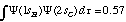where dt = dxdydz is the inifnitesimal volume element. The overlap integral between the hydrogen 1s orbital and the carbon 2pz orbital is 0.46, i.e.,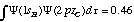The sp hybrid orbital that points along the z axis is a linear combination of the C 2s and 2pz orbitals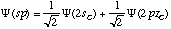whereas the sp3 orbital directed along the z axis is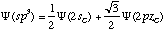Calculate the overlap integral between the hydrogen 1s and the carbon sp and sp3 hybrid orbitals directed along the z axis, which is taken to coincide with the C-H bond, assuming that the C-H bond distance of both ethyne and methane are the same. Does your result show the expected behavior? If not, can you suggest a reason for this failure?